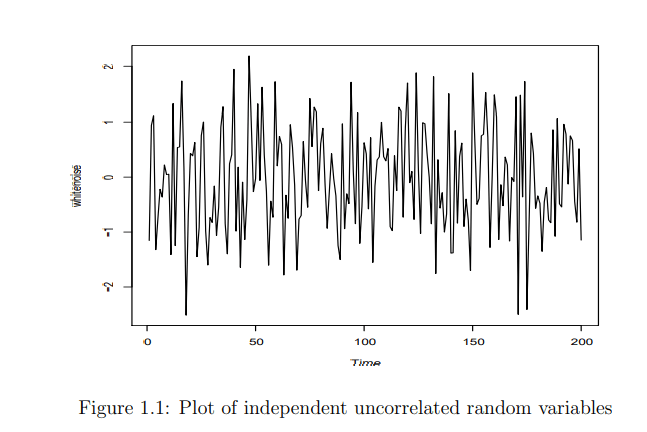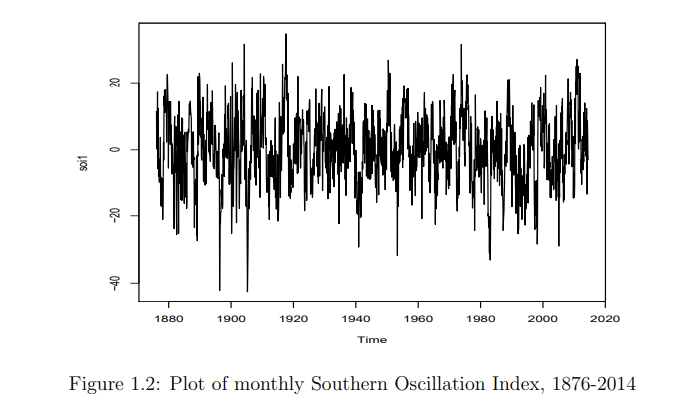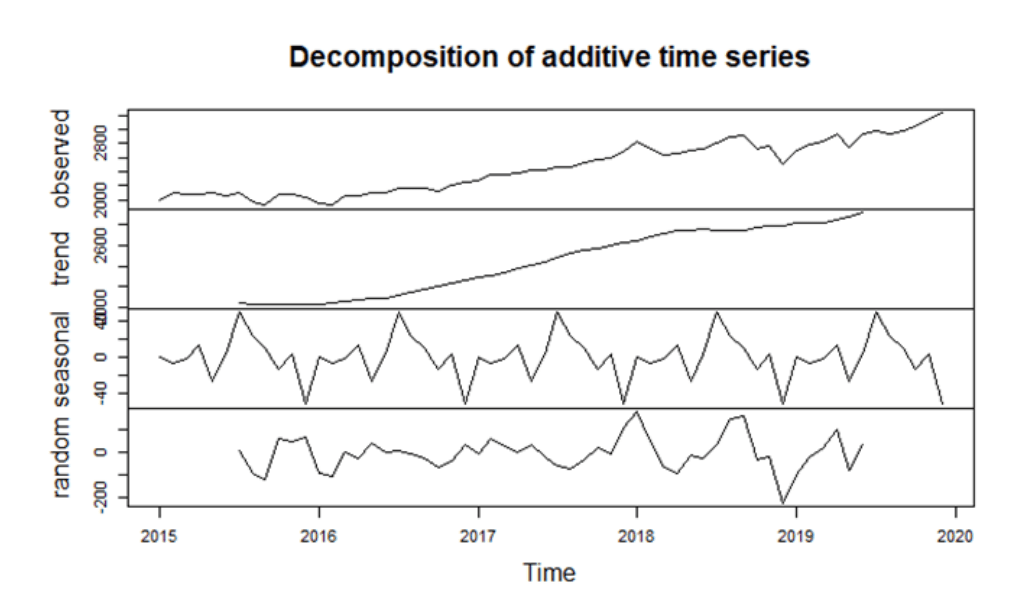## Definition

Time series analysis is a statistical technique that studies time series data. A time series is a sequence of data points indexed in discrete-time order.

A time series is a series of observations xt , observed over a period of time. Typically the observations can be over an entire interval, randomly sampled on an interval or at fixed time points. Different types of time sampling require different approaches to the data analysis.

## Time Series Data

The Southern Oscillation Index from 1876-present
The Southern Oscillation Index (SOI) is an indicator of intensity of the El Nino effect (see
wiki). The SOI measures the fluctuations in air surface pressures between Tahiti and Darwin.In Figure 1.2 we give a plot of monthly SOI from January 1876 – July 2014 (note that there is some doubt on the reliability of the data before 1930).## Univariate Time Series

A univariate time series is a stochastic (a fancy word for random) process that consists of a sequence of time indexed random variables 𝑋𝑡 = {𝑋1, 𝑋2, … }, where 𝑋1 denotes the random variable at time 𝑡 = 1, 𝑋2 the random variable at time 𝑡 = 2 and so on. The observed values of such a stochastic process, 𝑋1 = 𝑥1, 𝑋2 = 𝑥2 and so on is called a realization of the stochastic process. This sequence {𝑋𝑡} = {𝑥1, 𝑥2, … } is also called a time series. It will be clear from the context whether we are referring to the stochastic process or a particular realization of the process.

## Stationary processes

We have established that one of the main features that distinguish time series analysis from classical
methods is that observations taken over time (a time series) can be dependent and this dependency
tends to decline the further apart in time these two observations. However, to do any sort of analysis
of this time series we have to assume some sort of invariance in the time series, for example the mean
or variance of the time series does not change over time. If the marginal distributions of the time
series were totally different no sort of inference would be possible (suppose in classical statistics you
were given independent random variables all with different distributions, what parameter would you be estimating, it is not possible to estimate anything!).

## Types of stationarity

There are two definitions of stationarity, weak stationarity which only concerns the covariance of a
process and strict stationarity which is a much stronger condition and supposes the distributions
are invariant over time.

Definition 3.3.1 (Strict stationarity)

The time series {Xt} is said to be strictly stationary if for any finite sequence of integers t1, . . . , tk and shift h the distribution of (Xt1, . . . , Xtk) and (Xt1+h, . . . , Xtk+h) are the same.
The above assumption is often considered to be rather strong (and given a data it is very hard to check). Often it is possible to work under a weaker assumption called weak/second order stationarity.

Definition 3.3.2 (Second order stationarity/weak stationarity)

The time series {Xt} is said to be second order stationary if the mean is constant for all t and if for any t and k the covariance between Xt and Xt+k only depends on the lag difference k. In other words there exists a function
c : Z → R such that for all t and k we have
c(k) = cov(Xt, Xt+k).

# Covariance and Correlation:

## • Observe var(X) = cov(X, X).

• Rules of covariances. If a, b, c are finite constants and X, Y, Z are random variables with E(X2) < ∞, E(Y2) < ∞ and E(Z2) < ∞ (which immediately implies their means are finite). Then the covariance satisfies the linearity property

cov (aX + bY + c, Z) = acov(X, Z) + bcov(Y, Z)

Observe the shift c plays no role in the covariance (since it simply shifts the data).

• The variance of vectors. Suppose that A is a matrix and X a random vector with variance/- covariance matrix Σ. Then

var(AX) = Avar(X)A’ = AΣA

which can be proved using the linearity property of covariances.

• The correlation between X and Y is

cor(X, Y ) = cov(X, Y )/((var(X)var(Y ))^0.5)

and lies between [−1, 1]. If var(X) = var(Y ) then cor(X, Y ) is the coefficient of the best linear predictor of X given Y and visa versa.

## White Noise Process and Random Walk:

There is a special time series called white noise. Let {𝜀𝑡} be a white noise process, then
𝜀𝑡
satisfies

1. E(𝜀𝑡) = 𝜇 < ∞, ∀𝑡

2. var(𝜀𝑡) = 𝜎2 < ∞, ∀𝑡

3. cov(𝜀𝑡, 𝜀𝑠) = 0, ∀𝑡 ≠ s

A random walk is a stochastic process that is a sum of random steps. A summation of 𝜀𝑡 , 𝑊𝑡 = ∑ 𝜀𝑖 𝑡 𝑖=1 , is a random walk. Each next value 𝑊𝑡+1 is a random step away from the previous value. When E(𝜀𝑡) = 0, 𝑊𝑡 is called a random-walk-without-drift model. A Random Walk Down Wall Street suggests that the market is a random walk. This is consistent with the efficient-market hypothesis that past information does not help predicting the future.

## Ljung-Box Test for White Noise

The Ljung-Box test is a hypothesis test for zero autocorrelations for a time series or a series of residuals. It tests whether any in a group of autocorrelations of a time series is different from zero. The sample ACF 𝜌̂ are calculated for a number of lags that we want to test for. The null hypothesis is that the first 𝑚 autocorrelations are jointly zero.

## Model Decomposition

White noise process is very important in time series analysis. It is a fundamental building block of any time series. The Wold decomposition theorem says that for any covariance stationary stochastic process {𝑋𝑡}, it can be written as the sum of a deterministic time series and a stochastic time series. Mathematically, if {𝑋𝑡} is a
covariance stationary process and {𝜀𝑡} a white noise zero-mean process, then there exists a unique linear representation

𝑋𝑡 = 𝜇 + 𝑉𝑡 + 𝜀𝑡 + 𝜓1𝜀𝑡−1 + 𝜓2𝜀𝑡−2 + ⋯ = 𝜇 + 𝑉𝑡 + ∑𝜓𝑗𝜀𝑡−𝑗 (summation for j=0 to infi.)

The determinist component can be a trend or a seasonal component or both. In general,a time series can be decomposed into three components:
1. trend component
2. seasonal component
3. irregular random component

Trend component exists when there is a long-term increase or decrease in the data. For instance, S&P 500 increases with time. Seasonal component exists when the data exhibit patterns with seasons like months or quarters. For instance, the number of visitors to Disneyland changes with seasons (more people in summer and fewer in winter). Irregular random component, a.k.a. stochastic component or white noise, is the
innovations with time. That is the source of uncertainty of a time series. For instance, the return of the S&P 500 is an irregular process.

This decomposition is called the classical decomposition model. There are two forms of decomposition: additive and multiplicative. They combine trend component 𝑇𝑡, seasonal component 𝑆𝑡 and random component 𝜀𝑡 to build a time series model.

𝑋𝑡 = 𝑇𝑡 + 𝑆𝑡 + 𝜀t

The multiplicative model multiplies these components:

𝑋𝑡 = 𝑇𝑡 × 𝑆𝑡 × 𝜀t## Time Series Models

In general, we can use a function of time or a smoothing method (like moving average) to model the trend component. We can use a function of dummy variable(s) to model the seasonal component. After removing the trend and seasonal components, we are left with the random residuals. Below, we summarize the general steps of time series modeling.

1. Plot the time series to check for non-stationary behavior, such as trend and seasonal components.

2. Remove the non-stationary components by such as subtracting trend and seasonality, taking first difference and etc., to obtain a stationary time series of random residuals.

3. Choose an appropriate time series model to fit the residuals.

4. Check the goodness-of-fit of the model.

5. Invert the operations in step 2, such as adding back the trend and seasonal components, integrating the residuals and etc., to build a model for the original time series.

There are a number of important time series models to model the random residuals. They are the subjects of this section.

## AR, MA, ARMA, and ARIMA models

AR, MA, ARMA, and ARIMA models are used to forecast the observation at (t+1) based on the historical data of previous time spots recorded for the same observation. However, it is necessary to make sure that the time series is stationary over the historical data of observation overtime period. If the time series is not stationary then we could apply the differencing factor on the records and see if the graph of the time series is a stationary overtime period.

## ARMAX MODEL

An ARMAX model simply adds in the covariate on the right hand side:<span id="MathJax-Element-5-Frame" class="mjx-chtml MathJax_CHTML" style="margin: 0px;padding: 1px 0px;border: 0px;font-size: 17px;vertical-align: baseline;background: 0px 0px;line-height: 0;float: none;direction: ltr;max-width: none;max-height: none;min-width: 0px;min-height: 0px" role="presentation" data-mathml="“>

${y}_{t}=\beta {x}_{t}+{\varphi }_{1}{y}_{t-1}+\cdots +{\varphi }_{p}{y}_{t-p}-{\theta }_{1}{z}_{t-1}-\cdots -{\theta }_{q}{z}_{t-q}+{z}_{t}$

where <span id="MathJax-Element-6-Frame" class="mjx-chtml MathJax_CHTML" style="margin: 0px;padding: 1px 0px;border: 0px;font-size: 17px;vertical-align: baseline;background: 0px 0px;line-height: 0;float: none;direction: ltr;max-width: none;max-height: none;min-width: 0px;min-height: 0px" role="presentation" data-mathml="“>is a covariate at time <span id="MathJax-Element-7-Frame" class="mjx-chtml MathJax_CHTML" style="background: 0px 0px;margin: 0px;padding: 1px 0px;border: 0px;font-size: 17px;vertical-align: baseline;line-height: 0;float: none;direction: ltr;max-width: none;max-height: none;min-width: 0px;min-height: 0px" role="presentation" data-mathml="“> and <span id="MathJax-Element-8-Frame" class="mjx-chtml MathJax_CHTML" style="background: 0px 0px;margin: 0px;padding: 1px 0px;border: 0px;font-size: 17px;vertical-align: baseline;line-height: 0;float: none;direction: ltr;max-width: none;max-height: none;min-width: 0px;min-height: 0px" role="presentation" data-mathml="“> is its coefficient. While this looks straight-forward, one disadvantage is that the covariate coefficient is hard to interpret. The value of <span id="MathJax-Element-9-Frame" class="mjx-chtml MathJax_CHTML" style="background: 0px 0px;margin: 0px;padding: 1px 0px;border: 0px;font-size: 17px;vertical-align: baseline;line-height: 0;float: none;direction: ltr;max-width: none;max-height: none;min-width: 0px;min-height: 0px" role="presentation" data-mathml="“> is not the effect on <span id="MathJax-Element-10-Frame" class="mjx-chtml MathJax_CHTML" style="background: 0px 0px;margin: 0px;padding: 1px 0px;border: 0px;font-size: 17px;vertical-align: baseline;line-height: 0;float: none;direction: ltr;max-width: none;max-height: none;min-width: 0px;min-height: 0px" role="presentation" data-mathml="“>

when the <span id="MathJax-Element-11-Frame" class="mjx-chtml MathJax_CHTML" style="margin: 0px;padding: 1px 0px;border: 0px;font-size: 17px;vertical-align: baseline;background: 0px 0px;line-height: 0;float: none;direction: ltr;max-width: none;max-height: none;min-width: 0px;min-height: 0px" role="presentation" data-mathml="“>is increased by one (as it is in regression). The presence of lagged values of the response variable on the right hand side of the equation mean that <span id="MathJax-Element-12-Frame" class="mjx-chtml MathJax_CHTML" style="background: 0px 0px;margin: 0px;padding: 1px 0px;border: 0px;font-size: 17px;vertical-align: baseline;line-height: 0;float: none;direction: ltr;max-width: none;max-height: none;min-width: 0px;min-height: 0px" role="presentation" data-mathml="“> can only be interpreted conditional on the value of previous values of the response variable, which is hardly intuitive.

If we write the model using backshift operators, the ARMAX model is given                                 by<span id="MathJax-Element-13-Frame" class="mjx-chtml MathJax_CHTML" style="margin: 0px;padding: 1px 0px;border: 0px;font-size: 17px;vertical-align: baseline;background: 0px 0px;line-height: 0;float: none;direction: ltr;max-width: none;max-height: none;min-width: 0px;min-height: 0px" role="presentation" data-mathml="“>

$\varphi \left(B\right){y}_{t}=\beta {x}_{t}+\theta \left(B\right){z}_{t}\phantom{\rule{2em}{0ex}}\text{or}\phantom{\rule{2em}{0ex}}{y}_{t}=\frac{\beta }{\varphi \left(B\right)}{x}_{t}+\frac{\theta \left(B\right)}{\varphi \left(B\right)}{z}_{t},$

where <span id="MathJax-Element-14-Frame" class="mjx-chtml MathJax_CHTML" style="margin: 0px;padding: 1px 0px;border: 0px;font-size: 17px;vertical-align: baseline;background: 0px 0px;line-height: 0;float: none;direction: ltr;max-width: none;max-height: none;min-width: 0px;min-height: 0px" role="presentation" data-mathml="“>

ϕ(B)=1ϕ1BϕpB and <span id="MathJax-Element-15-Frame" class="mjx-chtml MathJax_CHTML" style="background: 0px 0px;margin: 0px;padding: 1px 0px;border: 0px;font-size: 17px;vertical-align: baseline;line-height: 0;float: none;direction: ltr;max-width: none;max-height: none;min-width: 0px;min-height: 0px" role="presentation" data-mathml="“>

$\theta \left(B\right)=1-{\theta }_{1}B-\cdots -{\theta }_{q}{B}^{\mathrm{q.}}$